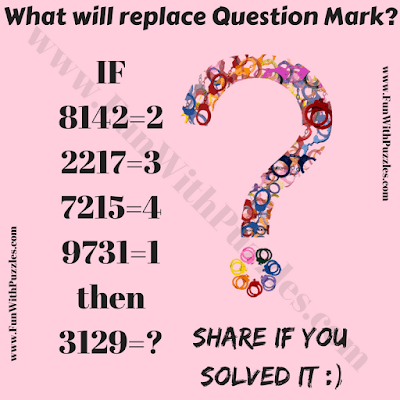Here is the test of your brain power. Let us see if you can solve this tough Maths Logic Question and find the value of the missing number. In this Puzzle Picture, there are some number equations given and your challenge is to find the relationship between the left side of the equation and the right side of the equation. So can you solve this tough Maths Logic Question Puzzle?Can you solve this tough Maths Logic Question?